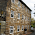## 2015/04/13

### Python筆記：反轉字串

s = 'Hello Python'

# s.reverse()

print(s[::-1])

print(''.join(reversed(s)))

def reverse_i(s):
r = ''
for c in s:
r = c + r
return r
print(reverse_i(s))

def reverse_r(s):
if len(s) <= 1:
return s
else:
return reverse_r(s[1:]) + s
print(reverse_r(s))

r = list(s)
r.reverse()
print(''.join(r))

def reverse_li(s):
r = []
for c in s:
r.insert(0, c)
return ''.join(r)
print(reverse_li(s))

print(''.join([s[i] for i in range(len(s)-1, -1, -1)]))

print(''.join(s[i] for i in range(len(s)-1, -1, -1)))

def reverse_g(s):
def sub(s):
for i in range(len(s)-1, -1, -1):
yield s[i]
return ''.join(sub(s))
print(reverse_g(s))

from collections import deque
r = deque(s)
r.reverse()
print(''.join(r))

print(s[::-1])
print(''.join(reversed(s)))

1.不好意思
我是python的初學者
請問一下迭代中的
r =‘ ’
For c in s:
c = c + r
是什麼意思呢

1.不是c = c + r，而是r = c + r。

假設s是'abc'，
迴圈第一輪，r會變成'a' + '' 也就是'a'，
迴圈第二輪，r會變成'b' + 'a' 也就是'ba'，
迴圈第二輪，r會變成'c' + 'ba' 也就是'cba'，

達到反轉字串的目的。

2.非常感謝清楚的說明